# Colleges with the highest SAT scores in Washington

Top 10 colleges in Washington with the highest SAT scores
Looking for the colleges with the highest SAT scores in Washington? Well you're in luck! We've compiled a national college database and have created a list of the top 10 universities with the highest SAT scores in Washington. These are the schools whose applicants had the highest average SAT scores in Washington. And, since these tests are meant to determine academic prowess, they are arguably the schools with the most academically proficient students. You could even say these are the best colleges in Washington. We also include each college's ACT scores and acceptance rate so that you can see where you would have the easiest or hardest time getting in. Read on to find out more.

## University of Washington SAT scores

The average SAT score for University of Washington is 1340.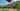The average SAT score of 1340 breaks down into:

• SAT math: 690

The average ACT score for University of Washington is 30 and their acceptance rate is 48.7%.

## DigiPen Institute of Technology SAT scores

The average SAT score for DigiPen Institute of Technology is 1272.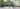The average SAT score of 1272 breaks down into:

• SAT math: 630

The average ACT score for DigiPen Institute of Technology is 27 and their acceptance rate is 57%.

## Gonzaga University SAT scores

The average SAT score for Gonzaga University is 1262.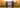The average SAT score of 1262 breaks down into:

• SAT math: 630

The average ACT score for Gonzaga University is 28 and their acceptance rate is 65.6%.

## University of Puget Sound SAT scores

The average SAT score for University of Puget Sound is 1237.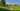The average SAT score of 1237 breaks down into:

• SAT math: 610

The average ACT score for University of Puget Sound is 28 and their acceptance rate is 88.3%.

## Seattle University SAT scores

The average SAT score for Seattle University is 1227.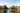The average SAT score of 1227 breaks down into:

• SAT math: 610

The average ACT score for Seattle University is 27 and their acceptance rate is 75.6%.

## Whitman College SAT scores

The average SAT score for Whitman College is 1200.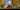The average SAT score of 1200 breaks down into:

• SAT math: 600

The average ACT score for Whitman College is 29 and their acceptance rate is 51.7%.

## Seattle Pacific University SAT scores

The average SAT score for Seattle Pacific University is 1190.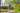The average SAT score of 1190 breaks down into:

• SAT math: 570

The average ACT score for Seattle Pacific University is 24 and their acceptance rate is 90.9%.

## Whitworth University SAT scores

The average SAT score for Whitworth University is 1190.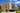The average SAT score of 1190 breaks down into:

• SAT math: 590

The average ACT score for Whitworth University is 25 and their acceptance rate is 90.8%.

## Western Washington University SAT scores

The average SAT score for Western Washington University is 1179.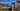The average SAT score of 1179 breaks down into:

• SAT math: 580

The average ACT score for Western Washington University is 25 and their acceptance rate is 87.7%.

## Pacific Lutheran University SAT scores

The average SAT score for Pacific Lutheran University is 1170.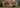The average SAT score of 1170 breaks down into:

• SAT math: 580